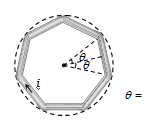NEET Physics Moving Charges and Magnetism Questions Solved

In the following figure a wire bent in the form of a regular polygon of n sides is inscribed in a circle of radius a. Net magnetic field at centre will be(a) $\frac{{\mu }_{o}i}{2\mathrm{\pi a}}\mathrm{tan}\frac{\mathrm{\pi }}{n}$                                                (b) $\frac{{\mu }_{0}ni}{2\mathrm{\pi a}}\mathrm{tan}\frac{\mathrm{\pi }}{n}$

(c)$\frac{2}{\mathrm{\pi }}\frac{ni}{a}{\mu }_{0}\mathrm{tan}\frac{\mathrm{\pi }}{n}$                                             (d) $\frac{ni}{2a}{\mu }_{0}\mathrm{tan}\frac{\mathrm{\pi }}{n}$

Explanation is a part of a Paid Course. To view Explanation Please buy the course.

Difficulty Level: